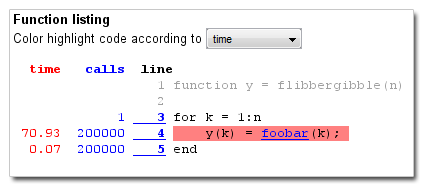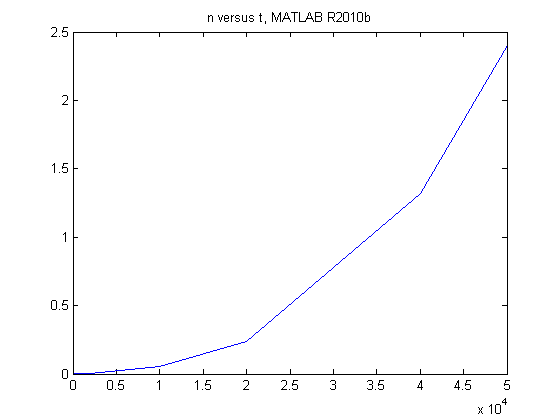# Automatic array growth gets a lot faster in R2011a

One of the most significant MATLAB improvements in the R2011a release is in how certain kinds of array growth operations are handled. This is a kind of "under-the-hood" change that I think is exciting and deserves some publicity.

For those of you who are MATLAB power users and understand preallocation and why it is necessary, I'll tell you the story's ending first: MATLAB R2011a performs much better than previous MATLAB versions when performing repeated array growth in a loop.

For everyone else, let's start with some not-so-hypothetical tech support questions:

• "Why is the MATLAB function foobar so slow?"
• "Why does MATLAB hang if I put a call to foobar in a loop?"
• "Why does the function foobar run so much faster at the command line than when I put it in a function?"

The tech support engineer will start digging into the question to see why the unhappy MATLAB user has reached his conclusion:

"Well, I ran the profiler on my function flibbergibble, which calls foobar:"You can see that foobar takes about 0.35 ms per call (70.93 / 200000), but when I call foobar 200000 times from the command line in a loop it only takes 8.5e-7 seconds per call:

 tic, for k = 1:200000, foobar(k); end, toc
Elapsed time is 0.173287 seconds.

"That's more than 400 times faster! Why is foobar so slow in a function?"

Experienced MATLAB users know that the performance problem has little to do with foobar(k) on line 4 of the profile report and much to do with y(k) = on the same line.

Let's examine why. The first time through the loop, the variable y hasn't been assigned to yet, so MATLAB creates it through the assignment to y(1). The second time through the loop assigns to y(2), which doesn't exist yet. So MATLAB allocates enough new memory for two elements and then copies y(1) and assigns y(2) into the new space. The third time through the loop assigns to y(3), which doesn't exist yet. So MATLAB allocates enough new memory for three elements and then copies y(1) and y(2) and assigns y(3) into the new space.

See the pattern? On the k-th time through the loop, MATLAB has to make a copy of k elements into newly allocated memory. The time required to copy k elements is proportional to k. The time required to execute the entire loop is therefore proportional to the sum of the integers from 1 to n, which is n*(n+1)/2.

Bottom line: memory copying during the assignment statement causes the time required to execute the loop to be proportional to n^2.

Let's see some data. I timed flibbergibble for various values of n using MATLAB R2010b. Here's the data:

n = [100 200 500 1000 2000 10000 20000 40000 50000];
t = [1.2e-4 2.7e-4 8.6e-4 2.4e-3 2.8e-3 5.8e-2 .24 1.32 2.4];

plot(n,t)
title('n versus t, MATLAB R2010b')That looks pretty parabola-like. I checked it out with cftool from the Curve Fitting Toolbox and found that a rough fit to the data is the curve 1.0e-9 * n^2. What if we tried n = 1000000? From our curve we could estimate that it would take about 17 minutes:

n = 1e6;
t_minutes = 1e-9 * n^2 / 60
t_minutes =

16.6667



This is what triggers the tech support call where the user thinks that the function foobar has caused MATLAB to hang. MATLAB isn't hung, it's just working really hard reallocating all that memory.

The solution is to preallocate space for y before beginning the loop, like this:

type flibbergibble2
function y = flibbergibble2(n)

y = zeros(1, n);
for k = 1:n
y(k) = foobar(k);
end



The code above does not have to reallocate any memory each time through the loop. In MATLAB R2010b on my computer it runs in less than a second:

 tic, flibbergibble2(1000000); toc
Elapsed time is 0.737451 seconds.

The big change in MATLAB R2011a is that MATLAB uses a better strategy for reallocating memory as the array grows when it detects this growth pattern. As a result, the time required to execute the loop grows only linearly with n.

Here's how it takes for n = 1000000 in MATLAB R2011a on my computer:

 tic, flibbergibble(1000000); toc
Elapsed time is 1.105256 seconds.

That's in the neighborhood of a thousand times faster than R2010b. You can see that the preallocation strategy (in flibbergibble2) is still a bit faster, but the performance penalty for not preallocating has been substantially reduced.

If the final size of your output array is trivial to calculate, then I would say go ahead and preallocate. It's just one extra code line and it's a little faster. But if the final size is complicated to calculate, then maybe you don't have to work as hard you did before to optimize your code. If you are growing a vector, or if the array growth is in the final dimension of a matrix or multidimensional array, you can now seriously consider just letting MATLAB do its own "automatic array growth" thing.

Published with MATLAB® 7.12

|

### コメント

コメントを残すには、ここ をクリックして MathWorks アカウントにサインインするか新しい MathWorks アカウントを作成します。